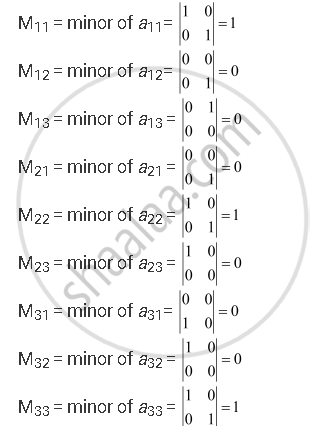Share

# Write Minors and Cofactors of the Elements of Following Determinants: |(1,0,0),(0,1,0),(0,0,1)| - CBSE (Commerce) Class 12 - Mathematics

#### Question

Write Minors and Cofactors of the elements of following determinants:

|(1,0,0),(0,1,0),(0,0,1)|

#### Solution

The given determinant is |(1,0,0),(0,1,0),(0,0,1)|

By the definition of minors and cofactors, we have:A11 = cofactor of a11= (−1)1+1 M11 = 1

A12 = cofactor of a12 = (−1)1+2 M12 = 0

A13 = cofactor of a13 = (−1)1+3 M13 = 0

A21 = cofactor of a21 = (−1)2+1 M21 = 0

A22 = cofactor of a22 = (−1)2+2 M22 = 1

A23 = cofactor of a23 = (−1)2+3 M23 = 0

A31 = cofactor of a31 = (−1)3+1 M31 = 0

A32 = cofactor of a32 = (−1)3+2 M32 = 0

A33 = cofactor of a33 = (−1)3+3 M33 = 1

Is there an error in this question or solution?

#### Video TutorialsVIEW ALL 

Solution Write Minors and Cofactors of the Elements of Following Determinants: |(1,0,0),(0,1,0),(0,0,1)| Concept: Minors and Co-factors.
S